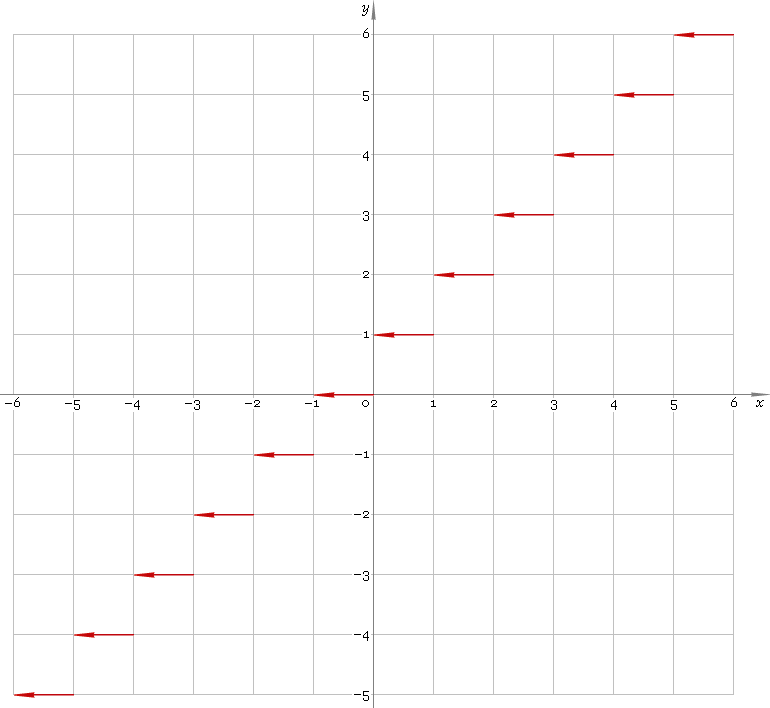The Art of Interface

# ceil — ceiling function

Category. Mathematics.

Abstract. Ceiling: definition, plot and properties.

## 1. Definition

Ceiling is the nearest integer to the righ — smallest integer greater than or equal to the argument.

## 2. Plot

Ceiling function defined everywhere on real axis — so, its domain is (−∞, +∞). Its stair-like plot is depicted below — fig. 1.Fig. 1. Plot of the ceiling function y = ceilx.

Function codomain is the set of integer numbers.

## 3. Properties

When using the function be aware, that in general case:

ceil(x) + ceil(y) ≠ ceil(x + y)
ceil(x) − ceil(y) ≠ ceil(xy)
ceil(x) ceil(y) ≠ ceil(x y)
ceil(x) /ceil(y) ≠ ceil(x /y)

## 4. Support

Ceiling function ceil of the complex argument is supported by professional version of the Librow calculator.

## 5. How to use

To calculate ceiling of the number:

``ceil(-1.8);``

To calculate ceiling of the current result:

``ceil(rslt);``

To calculate ceiling of the number x in memory:

``ceil(mem[x]);``